Browse by Topic
Related Topics

# 11/11/11 11:11:11—The Right Time to Look at a Number

World War I officially ended in 1918 at the 11th hour of the 11th day of the 11th month. Remembrance Day is observed in Commonwealth countries to recall the end of the war and to remember the members of the armed forces who gave their lives for the cause. This year we observe Remembrance Day on 11/11/11.

Beyond the somberness of this memorial day, those of us who are mathematically inclined consider the surprising ways we can combine 1s to achieve beautiful results. Some of these combinations involve rather unpleasant calculations, so we’ll let Mathematica do the heavy lifting while we marvel at the results!

Today I’ll share 11 interesting places in which 1 appears. Let’s jump in.

1. Can anything of interest come from combining the humble digit 1 with the square root and plus symbol? As the demonstration below suggests, given the right nesting, you get an infinite series that converges to φ = 1.61803…, the golden ratio.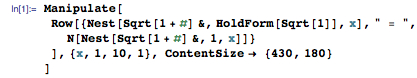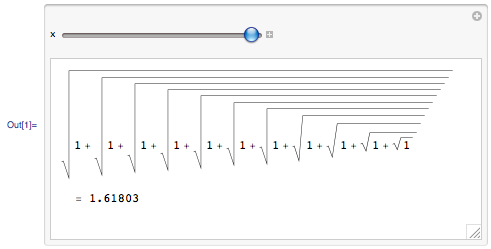2. Interestingly enough, a different combination of an infinite series of 1s also produces a series that converges to the golden ratio.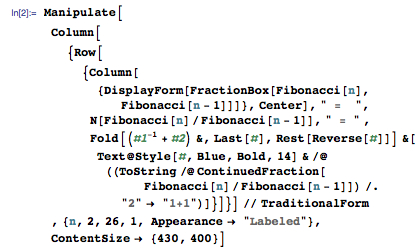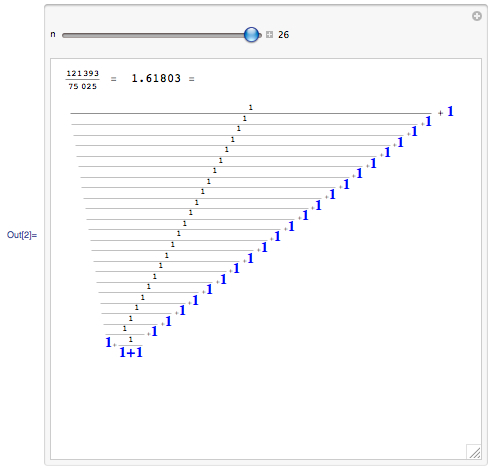3. I would be remiss if I didn’t find a way to include &#960 in this digits game. Here’s a series that (slowly) converges to &#960. Thank goodness that we don’t have to compute these products by hand!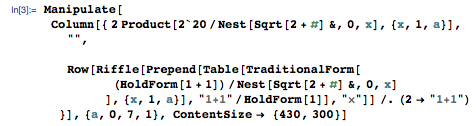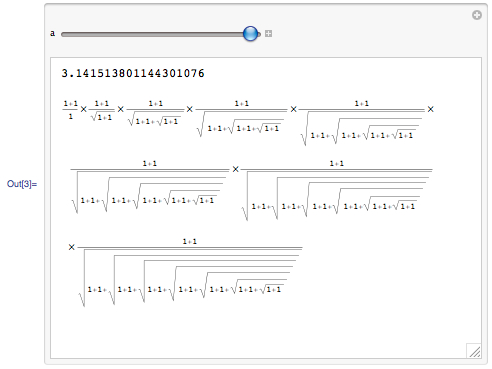4. Something interesting happens when we compute powers of 11. In particular, the digits of the first five powers of 11, through 114, correspond to the entries of the first five rows of Pascal’s triangle. (Can you see why this correspondence exists for these few terms and why it doesn’t exist beyond 114?)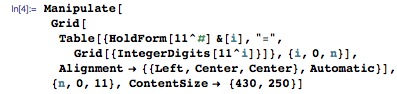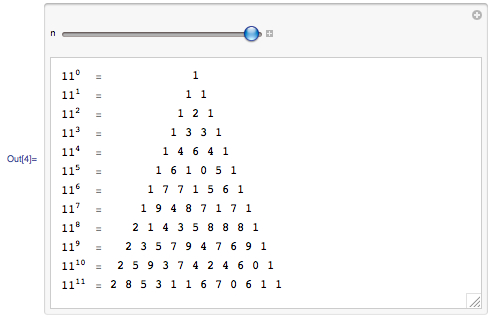5. What do you get when you multiply two numbers each consisting of all 1s? You get a pretty product.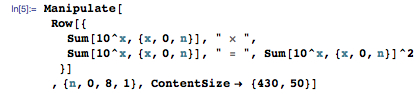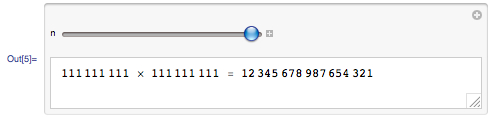6. Have you ever wondered what π(ππ) might be? In this expression, π is the number theory function (PrimePi in Mathematica) that tells how many primes there are that are less than or equal to a given number.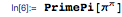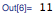7. How quickly can you compute the last 11 digits of 1111(11×11)? This number is so huge that it has more digits than the number of particles in the universe. Fortunately, Mathematica can quickly compute those last digits.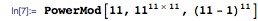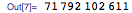8. Did you know that if a number is divisible by 11, then the number you get when you reverse its digits is also divisible by 11? Below is an example. (Note that FactorInteger[n] gives a list of the prime factors of the integer n together with their exponents.)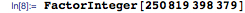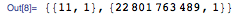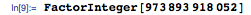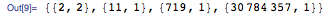9. What’s special about 11!+11!+11!+11!+11!+1? Yes, it’s prime.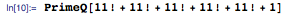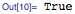10. Here’s a fun factoid that’s not at all related to Mathematica, but nonetheless worth including in any discussion about the number 11. Guess how many sides the polygon has that surrounds the portrait of Susan B. Anthony on the U.S. dollar coin? After you’ve answered that question, here’s a harder one. What do you call an 11-sided polygon? Hint: Wolfram|Alpha can help.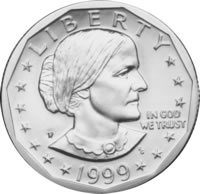11. Our final look at the digit 1 is an exploration. How many diagonals are there in a regular 11-sided polygon? How could you figure this out with and without Mathematica?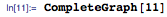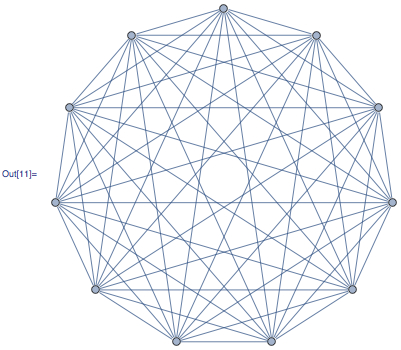I would like to thank the readers of Wild About Math! and the creators of the prime pages site for the suggestions, many of which inspired this article.